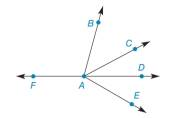Chapter 1.4, Problem 11E### Elementary Geometry for College St...

6th Edition
Daniel C. Alexander + 1 other
ISBN: 9781285195698

#### Solutions

Chapter
Section### Elementary Geometry for College St...

6th Edition
Daniel C. Alexander + 1 other
ISBN: 9781285195698
Textbook Problem
1 views

#Exercises 1 0 - 1 3 Without using a protractor, name the type of angle represented by: a) ∠ B A E b) ∠ F A D c) ∠ B A C d) ∠ F A E

To determine

a)

To find:

The type of the angle BAE.

Explanation

Definition:

If the measure of angle is greater than 90° and less than 180° then that angle is known as obtuse angle.

Approach:

The given angle is,

BAE

Consider the figure (1),

To determine

b)

To find:

The type of the angle FAD.

To determine

c)

To find:

The type of the angle BAC

To determine

d)

To find:

The type of the angle FAE.

### Still sussing out bartleby?

Check out a sample textbook solution.

See a sample solution

#### The Solution to Your Study Problems

Bartleby provides explanations to thousands of textbook problems written by our experts, many with advanced degrees!

Get Started

#### In Exercises 1-6, solve for y. x3y+2y=1

Calculus: An Applied Approach (MindTap Course List)

#### 2 1 0 does not exist

Study Guide for Stewart's Multivariable Calculus, 8th

#### The graph of x = 2 + 3t, y = 4 − t is a: circle ellipse line parabola

Study Guide for Stewart's Single Variable Calculus: Early Transcendentals, 8th